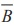Home | | Maths 11th std | Exercise 12.2: Some basic Theorems on Probability

# Exercise 12.2: Some basic Theorems on Probability

Maths Book back answers and solution for Exercise questions - Mathematics : Introduction to Probability Theory: Some basic Theorems on Probability

EXERCISE 12.2

(1) If A and B are mutually exclusive events P ( A) = 3/8 and P (B) = 1/8 , then(2) If A and B are two events associated with a random experiment for which P (A) = 0.35, P ( A or B) = 0.85, and P ( A and B) = 0.15.

Find (i) P (only B) (ii) P () (iii) P (only A)

(3) A die is thrown twice. Let A be the event, ŌĆśFirst die shows 5ŌĆÖ and B be the event, ŌĆśsecond die shows 5ŌĆÖ. Find P( A Ōł¬ B) .

(4) The probability of an event A occurring is 0.5 and B occurring is 0.3. If A and B are mutually exclusive events, then find the probability of(5) A town has 2 fire engines operating independently. The probability that a fire engine is available when needed is 0.96.

(i) What is the probability that a fire engine is available when needed?

(ii) What is the probability that neither is available when needed?

(6) The probability that a new railway bridge will get an award for its design is 0.48, the probability that it will get an award for the efficient use of materials is 0.36, and that it will get both awards is 0.2. What is the probability, that (i) it will get at least one of the two awards ( ii) it will get only one of the awards.

Answers Exercise 12.2 (1) (i) 5/8 (ii) 1/2 (iii)) 1/8 (iv) 1 (2) (i) 0.50 (ii) 0.35 (iii) 0.20 (3) 11/36 (4) (i) 0.8 (ii) 0.5 (iii) 0.3 (5) (i) 0.9984 (ii) 0.0016

(6) (i) 0.64 (ii) 0.44Tags : Problem Questions with Answer, Solution | Mathematics , 11th Mathematics : UNIT 12 : Introduction to Probability Theory
Study Material, Lecturing Notes, Assignment, Reference, Wiki description explanation, brief detail
11th Mathematics : UNIT 12 : Introduction to Probability Theory : Exercise 12.2: Some basic Theorems on Probability | Problem Questions with Answer, Solution | Mathematics